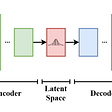# The Magic of Autoencoders

An auto-encoder is a sequential neural network, consisting of two components, the encoder and the decoder.

Let’s say we were dealing with images. Our encoder would extract features from the image which would reduce some components like its height and width, but makes a latent representation for the image. This latent representation just means the neural network only captures the most relevant characteristics of the input.

The decoder is the part of the neural network which learns how to reconstruct the data from the encoded version. The data is reconstructed in a way that it is as close to the original input as possible.

We also have a reconstruction loss which measures the performance of our decoder and how close the output data is to the original input data. To minimize loss, auto-encoders use backpropagation to minimize the neural network’s reconstruction loss.

Auto-encoders allow us to compress the data in an optimal way to reduce dimensions and ignore noise within the data.

Below is an example of how an autoencoder is used to encode and decode an image from the MIST dataset (handwritten digits). As you can see the original input and reconstructed input are very similar.

## Building an Autoencoder

I’ve built an auto-encoder in PyTorch, and I’ll show you step by step how you can build one too.

First, let's take an overview of the whole code:

`import torchimport torch.nn as nnfrom torchvision import datasetsfrom torch.autograd import Variablefrom torchvision.transforms import transforms#transform data to pytorch tensorstransforms = transforms.ToTensor()fashion_data = datasets.FashionMNIST(root='./data',  download=True, transform=transforms) #train=True,data_loader = torch.utils.data.DataLoader(fashion_data, batch_size=64, shuffle=True)#iterating through our datadataiter = iter(data_loader)images, labels = dataiter.next()#output will get the minimum tensor and the maximum tensor in the dataset --> important for our last activationprint(torch.min(images), torch.max(images))class autoencoder(nn.Module):    def __init__(self, epochs=10, batchSize=128, learningRate=1e-3, weight_decay=1e-5):        super(autoencoder, self).__init__()        self.epochs = epochs        self.batchSize = batchSize        self.learningRate = learningRate        self.weight_decay = weight_decay        #encoder        self.encoder = nn.Sequential(            nn.Linear(28 * 28, 128),  # reduces from n * 724 to 128            nn.ReLU(),            nn.Linear(128, 64),            nn.ReLU(),            nn.Linear(64, 12),            nn.ReLU(),            nn.Linear(12, 3)        )        #decoder        self.decoder = nn.Sequential(            nn.Linear(3, 12),            nn.ReLU(),            nn.Linear(12, 64),            nn.ReLU(),            nn.Linear(64, 128),            nn.ReLU(),            nn.Linear(128, 28 * 28),            nn.Sigmoid()  # cause tensors are 0, 1        )        self.optimizer = torch.optim.Adam(self.parameters(), lr=self.learningRate, weight_decay=self.weight_decay)        self.loss = nn.MSELoss()    #feed data through network    def forward(self, x):        encoder = self.encoder(x)        decoder = self.decoder(encoder)        return decoder    #training loop    def train(self):        for epoch in range(self.epochs):            for data in data_loader:                img, _ = data                img = img.view(img.size(0), -1)                img = Variable(img)                #predict                output = self(img)                # find loss                loss = self.loss(output, img)                # perform back propagation                self.optimizer.zero_grad()                loss.backward()                self.optimizer.step()            print(f'epoch {epoch + 1}/{self.epochs}, loss: {loss.data:.4f}')model = autoencoder()model.train()`

To break things down, let’s start off with our imports:

`import torchimport torch.nn as nnfrom torchvision import datasetsfrom torch.autograd import Variablefrom torchvision.transforms import transforms`

import torch → PyTorch module.

import torch.nn as nn → used for our neural network class.

from torchvision import datasets → allows us to use preloaded datasets in PyTorch.

from torch.autograd import Variable → we use a variable to store the value computed by a loss function. Variables have many functions related to tensors and backpropagation.

from torchvision.transforms import transforms → used for image preprocessing.

Ok, now that we have our imports, we can jump into getting and going through our data:

`#transform data to pytorch tensorstransforms = transforms.ToTensor()fashion_data = datasets.FashionMNIST(root='./data',  download=True, transform=transforms) #train=True,data_loader = torch.utils.data.DataLoader(fashion_data, batch_size=64, shuffle=True)#iterating through our datadataiter = iter(data_loader)images, labels = dataiter.next()`

First, we are going to initialize a variable called transforms, which when passed into the transform parameter, will transform an image into a PyTorch tensor.

`transforms = transforms.ToTensor()`

Next, we are going to create our fashion_data variable, which will use datasets.FashionMNIST to get the FashionMNIST data which is preloaded in PyTorch. We will also pass in our transforms variable into the transform parameter because we want to transform our images into tensors.

`fashion_data = datasets.FashionMNIST(root='./data', train=True, download=True, transform=transforms)`

PyTorch has a beautiful class called a DataLoader, which allows us to load data, and iterate over the elements, making life 10x as opposed to manually feeding in all the data.

Below, we have created a data_loader variable that uses PyTorch’s DataLoader to iterate over our fashion_data, giving it a batch size of 64 and shuffling the data.

`data_loader = torch.utils.data.DataLoader(fashion_data, batch_size=64, shuffle=True)`

Lastly, we are going to iterate through our data_loader, going through each image and label.

`#iterating through our datadataiter = iter(data_loader)images, labels = dataiter.next()`

We are going to print torch.min() of our images to find the minimum value for all the elements in the input tensor and torch.max() to find the maximum value for all the elements in the input tensor.

`#output will get the minimum tensor and the maximum tensor in the dataset --> important for our last activationprint(torch.min(images), torch.max(images))`

This last statement is important because the tensor values that are returned will determine what function we will need to use in the last layer of our decoder. Since the output shows our minimum tensor is tensor(0.) and the maximum tensor is tensor(1.), that means all the tensors will be between those numbers so we have to use the Sigmoid activation.

Remember → The sigmoid activation function is continuous and the derivative at all points always produces an output between the number 0 and 1.

Now that we’ve gotten our data ready and have learned some useful information, we can get to building our auto-encoder neural network.

Here is an overview of our whole code:

`class autoencoder(nn.Module):    def __init__(self, epochs=10, batchSize=128, learningRate=1e-3, weight_decay=1e-5):        super(autoencoder, self).__init__()        self.epochs = epochs        self.batchSize = batchSize        self.learningRate = learningRate        self.weight_decay = weight_decay        #encoder        self.encoder = nn.Sequential(            nn.Linear(28 * 28, 128),  # reduces from n * 724 to 128            nn.ReLU(),            nn.Linear(128, 64),            nn.ReLU(),            nn.Linear(64, 12),            nn.ReLU(),            nn.Linear(12, 3)        )        #decoder        self.decoder = nn.Sequential(            nn.Linear(3, 12),            nn.ReLU(),            nn.Linear(12, 64),            nn.ReLU(),            nn.Linear(64, 128),            nn.ReLU(),            nn.Linear(128, 28 * 28),            nn.Sigmoid()  # cause tensors are 0, 1        )        self.optimizer = torch.optim.Adam(self.parameters(), lr=self.learningRate, weight_decay=self.weight_decay)        self.loss = nn.MSELoss()    #feed data through network    def forward(self, x):        encoder = self.encoder(x)        decoder = self.decoder(encoder)        return decoder    #training loop    def train(self):        for epoch in range(self.epochs):            for data in data_loader:                img, _ = data                img = img.view(img.size(0), -1)                img = Variable(img)                #predict                output = self(img)                # find loss                loss = self.loss(output, img)                # perform back propagation                self.optimizer.zero_grad()                loss.backward()                self.optimizer.step()            print(f'epoch {epoch + 1}/{self.epochs}, loss: {loss.data:.4f}')model = autoencoder()model.train()`

Okay, this is a lot to take in so let’s break it down chunk by chunk :)

`class autoencoder(nn.Module):    def __init__(self, epochs=10, batchSize=128, learningRate=1e-3, weight_decay=1e-5):        super(autoencoder, self).__init__()        self.epochs = epochs        self.batchSize = batchSize        self.learningRate = learningRate        self.weight_decay = weight_decay        #encoder        self.encoder = nn.Sequential(            nn.Linear(28 * 28, 128),  # reduces from n * 724 to 128            nn.ReLU(),            nn.Linear(128, 64),            nn.ReLU(),            nn.Linear(64, 12),            nn.ReLU(),            nn.Linear(12, 3)        )        #decoder        self.decoder = nn.Sequential(            nn.Linear(3, 12),            nn.ReLU(),            nn.Linear(12, 64),            nn.ReLU(),            nn.Linear(64, 128),            nn.ReLU(),            nn.Linear(128, 28 * 28),            nn.Sigmoid()  # cause tensors are 0, 1        )        self.optimizer = torch.optim.Adam(self.parameters(), lr=self.learningRate, weight_decay=self.weight_decay)        self.loss = nn.MSELoss()`

We are going to call our class autoencoder, and it will inherit the nn.Module class. This is usually the base class for all neural nets in PyTorch.

To explain this module simply, it uses tensors and automatic differentiation modules (techniques to evaluate the derivative of a function) for training and building layers (input, hidden, output, etc).

In our __init__, we will pass in our epochs, batch size and learning rate, and initialize them. You can play around with the numbers, however, these are the numbers I found to work best.

`class autoencoder(nn.Module):    def __init__(self, epochs=10, batchSize=128, learningRate=1e-3, weight_decay=1e-5):        super(autoencoder, self).__init__()        self.epochs = epochs        self.batchSize = batchSize        self.learningRate = learningRate        self.weight_decay = weight_decay`

After our initialization, we can start off with building our encoder.

We will use a simple sequential structure with a linear layer followed by a relu activation. Notice that we pass in our image size as 28 by 28 because the fashion MNIST dataset has images that are 784 pixels. Every layer, we will decrease our input.

You can think of our input as N , 784 and the output of our encoder will dramatically reduce the size to N, 3.

`#encoderself.encoder = nn.Sequential(    nn.Linear(28 * 28, 128),  # reduces from n * 724 to 128    nn.ReLU(),    nn.Linear(128, 64),    nn.ReLU(),    nn.Linear(64, 12),    nn.ReLU(),    nn.Linear(12, 3))`

Our decoder has a similar structure to our encoder, however, we are going in the opposite direction which is → N,3 to N, 784.

For our last layer, notice we use the sigmoid activation (explained earlier).

`#decoderself.decoder = nn.Sequential(    nn.Linear(3, 12),    nn.ReLU(),    nn.Linear(12, 64),    nn.ReLU(),    nn.Linear(64, 128),    nn.ReLU(),    nn.Linear(128, 28 * 28),    nn.Sigmoid()  # cause tensors are 0, 1)`

Now we can initialize our optimizer and our criterion. Always make sure to define this below your auto-encoder or you won't be able to pass anything in.

Remember → Optimizers are algorithms that change the attributes belonging to your neural networks, such as weights and learning rate, to reduce the losses.

For our network, we will use the Adam optimizers. We will need to pass in self.parameters, our learning rate and our weight_decay (which we initialized earlier in our network).

Remember → the learning rate controls how quickly a network is adapted to a problem.

Remember → Regularization is a way to constraint our network to fit our data accurately, avoiding overfitting. Weight_decay is a way to perform regularization on a neural network.

`self.optimizer = torch.optim.Adam(self.parameters(), lr=self.learningRate, weight_decay=self.weight_decay)self.loss = nn.MSELoss()`

Okay, now we can feed our data into the network. We will take in x, pass it into our encoder, pass in our encoder into the decoder, and finally return the result of our decoder.

`#feed data through networkdef forward(self, x):    encoder = self.encoder(x)    decoder = self.decoder(encoder)    return decoder`

Now that we’ve built our network, we can create a function to train our model.

We will iterate through our dataset for every epoch and augment our images to fit the network. We will call predictions, calculate the loss, and then perform backpropagation. After every epoch, we will output the loss.

`#training loopdef train(self):    for epoch in range(self.epochs):        for data in data_loader:            img, _ = data            img = img.view(img.size(0), -1)            img = Variable(img)            #predict            output = self(img)            # find loss            loss = self.loss(output, img)            # perform back propagation            self.optimizer.zero_grad()            loss.backward()            self.optimizer.step()        print(f'epoch {epoch + 1}/{self.epochs}, loss: {loss.data:.4f}')`

Lastly, to run our model, we need to create an object of our autoencoder class, and we will call this object model. To train the model, we will call our train function on the object.

`model = autoencoder()model.train()`

I hope you enjoyed this explanation and tutorial on how to build an autoencoder in PyTorch! This model trains well with little loss, and you can test out the results by picking a specific image from the fashion MNIST, feeding it into the model and plotting the before and after results.

# Contact me for any inquiries 🚀

Hi, I’m Ashley, a 16-year-old coding nerd, and an A.I. and neuroscience enthusiast!

I hope you enjoyed reading my article, and if you did, feel free to check out some of my other pieces on Medium :)

The repository for this code is on my GitHub under my “Pytorch” repository.

Articles you will like if you read this one:

If you have any questions, would like to learn more about me, or want resources for anything A.I. or programming related, you can contact me by:

💫Email: ashleycinquires@gmail.com

--

--

--

## More from MLearning.ai

Data Scientists must think like an artist when finding a solution when creating a piece of code. ⚪️ Artists enjoy working on interesting problems, even if there is no obvious answer ⚪️ linktr.ee/mlearning 🔵 Follow to join our 18K+ Unique DAILY Readers 🟠

## AWS ML Stack Reduces the Undifferentiated Heavy-Lifting in Machine Learning Lifecycle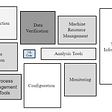## The SVM we need to know || The SVM we implemented.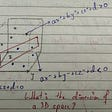## Designing an end-to-end Machine Learning Framework Using DataBricks MLFlow, Apache Airflow and AWS…## Humans overfit as well## Bisecting K-Means Algorithm — Clustering in Machine Learning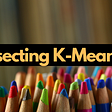## Face recognition for superimposed facemasks using VGGFace2 in Keras## Parametric VS Non-Parametric Methods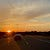## Ashley C

All things AI and emerging tech :)

## Organizing a Mess With Computer Vision## Classification of histological images with clear cell renal cell carcinoma, using Keras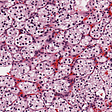## Deep Learning for Sign Language Production and Recognition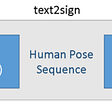## Variational Autoencoders